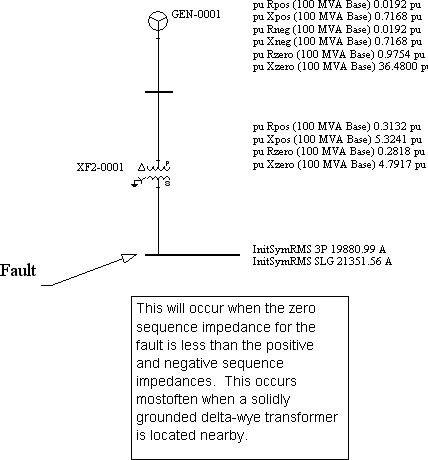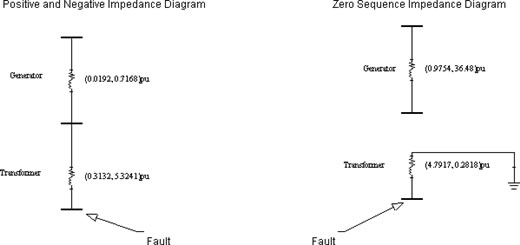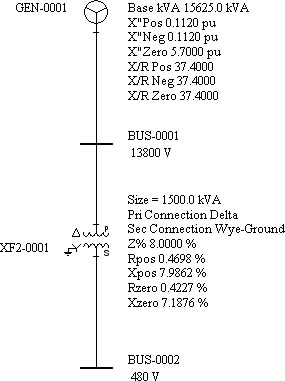CEE Relays Ltd

Tel:   +44 1753 576477    Fax: +44 1753 825661
FAQs: High Phase-Earth Short Circuit Currents
In most cases the 3-phase short-circuit current is higher than the phase-earth (SLG) short-circuit current. However, this is not always the case. This occurs when the zero-sequence impedance for the fault is less than the positive and negative impedances, such as when the fault is close to a solidly-earthed delta-star transformer, as shown below:Given Data:

Generator:

Size: 15625 KVA
X"pos = 0.1120pu       X/R = 37.4      Rpos-> = 0.00299pu
X"neg = .01120pu       X/R = 37.4      Rneg-> = 0.00299pu
X"zero = 5.70pu           X/R = 37.4      Rzero-> = 0.1524pu

Transformer:
Size: 1500 KVA 13800V/480V D/YG

Rpos = 0.004698 pu        Xpos = 0.079862pu
Rneg = 0.004698 pu        Xneg = 0.079862pu
Rzero = 0.004227pu        Xzero = 0.071876pu

Converting the impedance to 100MVA we get the following:

Zpu(new) = Zpu(old) (Sbase(new)/Sbase(old))(Vbase(old)/Vbase(new))2

Generator:
X"pos = 0.1120pu(100/15.625)(1.0) = 0.7168pu    Rpos = 0.00299pu(100/15.625)(1.0) = 0.0192pu
X"neg = 0.1120pu(100/15.625)(1.0) = 0.7168pu    Rneg = 0.00299pu(100/15.625)(1.0) = 0.0192pu
X"zero = 5.70pu (100/15.625)(1.0) = 36.48pu         Rzero = 0.1524pu (100/15.625)(1.0) = 0.9754pu

Transformer:
Rpos = 0.004698 pu(100/1.5)(1.0) = 0.3132pu      Xpos = 0.079862pu(100/1.5)(1.0) = 5.3241pu
Rneg = 0.004698 pu(100/1.5)(1.0) = 0.3132pu      Xneg = 0.079862pu(100/1.5)(1.0) = 5.3241 pu
Rzero = 0.004227pu(100/1.5)(1.0) = 0.2818pu      Xzero = 0.071876pu(100/1.5)(1.0) = 4.7917 puSince the transformer is solidly earthed, the only zero-sequence impedance component is from the transformer itself.

Generator zero-sequence impedance has been calculated for completeness although it does not contribute to the short circuit current.
Z+/-thev = (0.0192, 0.7168)pu + (0.3132, 5.3241)pu
= (0.3324, 6.0409)pu = (6.05 ∠86.85o)pu

Z0thev = (4.7917, 0.2818)pu = (4.799 ∠86.63o)pu

Ibase @ 480V
I = S/V√3 = 100MVA / 480 √3 = 120281.31 A

Calculating the fault current we get:

I3phase(pu) = E / Z+
= 1 / (6.05 ∠86.85o) = (0.165∠-86.85o

I3phase(actual) = (0.165∠-86.85o) x 120281.31 A = (19881.1∠-86.85o) A

Islg(pu) = 3E / (Z+ + Z- + Z0
= 3E / [(6.05 ∠86.85o)pu + (6.05 ∠86.85o)pu + (4.799 ∠86.63o)pu] =
= 3 / (16.9∠86.85o)pu
= (0.1775∠86.85o)pu

Islg(actual) = (0.1775∠86.85o)pu x 120281.31 A = (21351.67∠-86.80o) AComparing the magnitude of I3phase(actual) and Isgl(actual) shows that the single-phase fault current is actually higher than the three-phase fault current.